﻿ 基于LabVIEW的电能质量检测的应用

基于LabVIEW的电能质量检测的应用Application of Power Quality Detection Based on LabVIEW

Abstract: Good quality power is the guarantee of normal operation of power equipment. This paper intro-duces a real-time electric energy detection system based on LabVIEW. On the premise of using LabVIEW to analyze, a virtual instrument detection device for signal processing and data analysis is designed. The voltage and current parameters collected in real-time can be accurately displayed by a special module. Power quality analysis system based on virtual instrument is established.

1. 技术背景

2. 系统总体设计

2.1. 系统实现功能

1) 对电网的电压、电流波形的实时采集；

2) 使用Labview中自带的算法实现对波形的谐波分析，计算功率因数；

3) 使用RS485串口，实现上传数据，并通过Labview，将检测的数据显示出来。检测平台能够对电能自动检测系统的测试状态进行控制。

2.2. 系统总体方案设计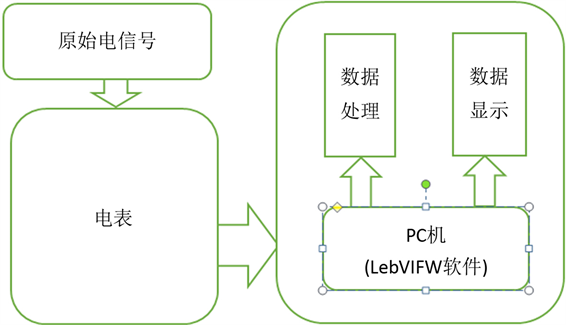Figure 1. Overall scheme design of the system

3. 系统总体模块设计

3.1. 数据采集模块设计Figure 2. Design of data acquisition module

3.2. 数据传输模块设计

RS485兼容TTL电平，可以方便的与TTL电路相连。在电平转换的设计中，采用芯片HYM3085将UART串行接口输出的TTL电平转换成差分信号，使其可以与外部RS485设备进行通信。本项目对典型方法进行优化设计，将RS485中的使能信号与DI (数字输入信号)分别控制的方式简化为用DI代替使能控制信号  。

1) 每秒传送的位数，例如4500表示每一秒传送的位数为4500个。

2) 数据位，指需要传送的真正内容，例如设为7，则每一帧数据中含有7位是真正需要发送的数据。

3) 停止位，串口通信时在每一帧的最后面加一个停止位表示本帧数据发送完毕，使得两台通信设备出现小的不同步时，能够自动校正。

4) 奇偶校验位，是用来验证收到的数据是否正确，具体来说是根据数据位0或者1的个数来决定校验位补1还是补0，奇校验时1的个数为奇数，偶校验时1的个数为偶数。

5) 流控制，指串口通信时使得发送与接收两端的协调的控制方式，也可以称作是两个通信端之间的握手。

3.3. USB串口通信模块

1) 通讯的第一步是根据资源的名称打开USB设备连接通过VISA的写入函数来实现向连接的USB设备发送控制指令；通过VISA的读入函数实现USB设备数据传输。根据USB的传输协议，通过不同的端点来接收采集到的数据及发送或读取控制命令。

2) 串口信息的读取与LabVIEW程序设计底层的I/O软件同顶层应用软件之间是通过设备驱动程序作为中介联系在一起的。在本系统中驱动程序是直接采用N提供的VISA自动生成的，从而最终实现了LABVEW的USB通讯功能  。

3.4. LabVIEW程序设计模块

3.4.1. 电压、电流的测量

$U=\sqrt{\frac{{\int }_{0}^{T}{U}^{2}\left(t\right)\text{d}t}{T}}\text{\hspace{0.17em}}\text{\hspace{0.17em}}\text{\hspace{0.17em}}I=\sqrt{\frac{{\int }_{0}^{T}{i}^{2}\left(t\right)\text{d}t}{T}}$

${U}_{rms}=\sqrt{\frac{{\sum }_{n=1}^{N}{u}^{2}\left(n\right)}{N}}\text{\hspace{0.17em}}\text{\hspace{0.17em}}\text{\hspace{0.17em}}{I}_{rms}=\sqrt{\frac{{\sum }_{n=1}^{N}{i}^{2}\left(n\right)}{N}}$

3.4.2. 功率测量算法

$P=\frac{1}{T}{\int }_{0}^{T}u\left(t\right)i\left(t\right)\text{d}t$

$P=\frac{1}{N}{\sum }_{n=1}^{N}u\left(n\right)i\left(n\right)$

4. 方案效果

LabVIEW可实现两大电能参数数据的显示：1、实现仪表数据的传输和显示2、模拟电路的传输和显示(通过正弦波模拟系统实现)。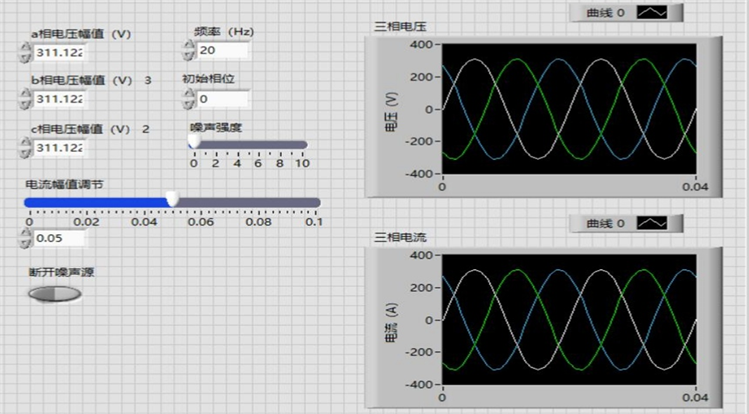Figure 3. Real-time front panel acquisition of three-phase voltage and current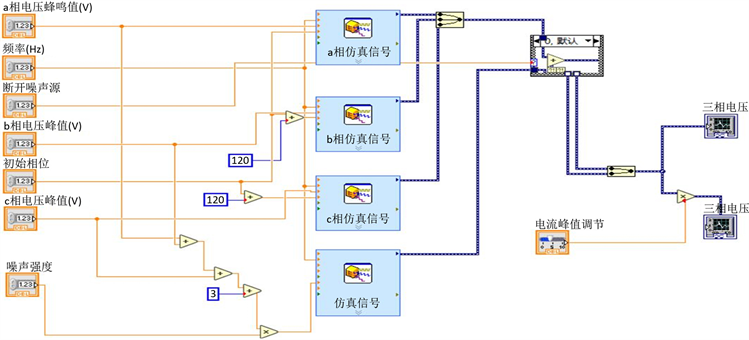Figure 4. Program block diagram of three-phase current and voltage simulation signal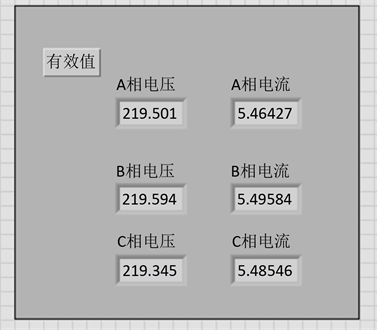Figure 5. Three-phase voltage and current RMS front panel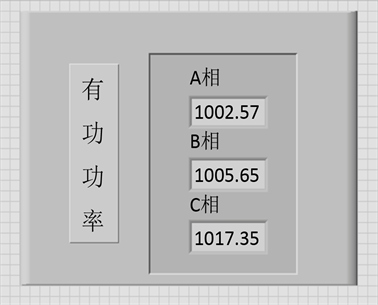Figure 6. Active power measurement front panel

5. 总结

 王铎. 基于LabVIEW电能质量监测系统的研究[D]: [硕士学位论文]. 长春: 吉林大学, 2013.

 李建国, 陈永超, 赖立海, 刘建民. 基于LabVIEW和RS485通信的光伏监测系统[J]. 自动化与仪表, 2014, 29(9): 16-19.

 陈锡辉, 张银鸿, 著. LABVIEW 8.20程序设计从入门到精通[M]. 北京: 清华大学出版社, 2007.

 刘勇平. 基于LabVIEW的电能质量监测实验平台设计[D]: [硕士学位论文]. 太原: 太原科技大学, 2015.

 邢磊. 电能质量检测系统的研究与设计[D]: [硕士学位论文]. 成都: 西华大学, 2013.

Top